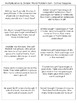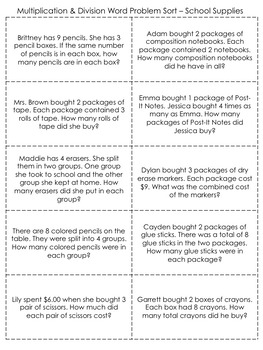# Free Sample - Multiply or Divide?: A Word Problem SortSubject
Resource Type
File Type

PDF

(6 MB|10 pages)
Standards
• Product Description
• Standards
Choosing the correct operation when solving a word problem is a struggle for many students. I created word problem sorts to help students focus on the operation needed. When completing the sort they have to slow down and make a decision before solving.

In this activity students must distinguish between division word problems and multiplication word problems. Students create a T chart with division on one side and multiplication on the other side. Students cut out each word problem and glue it on the correct side of their T chart. Then they write an equation and solution to the problem. You can have students make these into posters on construction paper or put them in interactive notebooks.

This free product is a sample of my "Multiply or Divide?" word problem sort containing 7 additional sorts. Purchase the full product here .

Standards:
• Represent and solve problems involving multiplication and division.
• Interpret products and quotients of whole numbers.
• Use multiplication and division within 100 to solve word problems.

What Teachers are Saying:
Thank you for the practice. Students often have a difficult time when you begin mixing operations! - Pamela H.
• I love how this makes students really think! Thank you for sharing your work! - Cheri H.
• Thanks so much! This is seriously cool! I will use in our intensive needs unit. - Kathleen F.

Full version of 8 differentiated sorts:
Multiply or Divide - Differentiated Word Problem Sorts

Related Products:
Add or Multiply? Word Problem Sort Free Sample
Add or Subtract? Word Problem Sort Free Sample

Permission to copy for one classroom teacher.
Electronic distribution for one classroom teacher.

Interpret whole-number quotients of whole numbers, e.g., interpret 56 ÷ 8 as the number of objects in each share when 56 objects are partitioned equally into 8 shares, or as a number of shares when 56 objects are partitioned into equal shares of 8 objects each. For example, describe a context in which a number of shares or a number of groups can be expressed as 56 ÷ 8.
Interpret products of whole numbers, e.g., interpret 5 × 7 as the total number of objects in 5 groups of 7 objects each. For example, describe a context in which a total number of objects can be expressed as 5 × 7.
Total Pages
10 pages
Included
Teaching Duration
40 minutes
Report this Resource to TpT
Reported resources will be reviewed by our team. Report this resource to let us know if this resource violates TpT’s content guidelines.